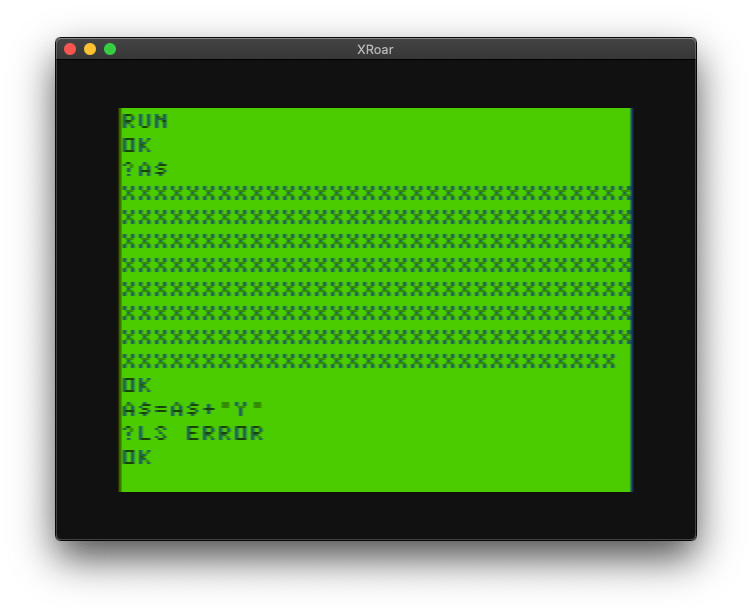# Color BASIC and string concatenation

The C programming language has a few standard library functions that deal with strings, namely strcpy() (string copy) and strcat() (string concatenate).

Microsoft BASIC has similar string manipulation features built in to the language. For example, to copy an 8 character “string” in to a buffer (array of chars):

``````char buffer1;

strcpy(buffer1, "12345678");

printf("%s\n", buffer1);``````

In BASIC, you do not need to allocate space for individual strings. Color BASIC allows doing whatever you want with a string provided it is 255 characters or less, and provided the total string space is large enough. By default, Color BASIC reserves 200 bytes for string storage. If you wanted to strcpy() “12345678” to a string variable, you would just do:

``BUFFER1\$="12345678"``

Yes, that’s legal, but Color BASIC only recognizes the first two characters of a string name, so in reality, that is just like doing:

`BU\$="12345678"`

If you need more than the default 200 bytes, the CLEAR command will reserve more string storage. For example, “CLEAR 500” or “CLEAR 10000”.

“CLEAR 500” would let you have five 100 character strings, or 500 one character strings.

And, keep in mind, strings stored in PROGRAM MEMORY do not use this space. For example, if you reduced string space to only 9 bytes, then tried to make a 10 byte string direct from BASIC:

```CLEAR 9
A\$="1234567890"
?OS ERROR```

The wonderful “?OS ERROR” (Out of String Space).

BUT, if strings are declared inside PROGRAM data, BASIC references them from within your program instead of string memory:

``````5 CLEAR 9
10 A\$="1234567890"
20 B\$="1234567890"
30 C\$="1234567890"
40 D\$="1234567890"
50 E\$="1234567890"``````

Yes, that actually works. If you would like to know more, please see my String Theory series.

But I digress…

The other common C string function is strcat(), which appends a string at the end of another:

```char buffer1;

strcpy(buffer1, "12345678");
strcat(buffer1, "plus this");

printf("%s\n", buffer1);```

That code would COPY “12345678” in to the buffer1 memory, then concatenate “plus this” to the end of it, printing out “12345678plus this”.

In BASIC, string concatenation is done by adding the strings together, such as:

```A\$="12345678"
A\$=A\$+"plus this"

PRINT A\$```

Color BASIC allows for strings to be up to 255 characters long, and no more:

The wonderful “?LS ERROR” (Length of String).

## Make it Bigger!

In something I am writing, I started out with an 8 character string, and wanted to duplicate it until it was 64 characters long. I did it like this:

```10 A\$="12345678"
20 A\$=A\$+A\$+A\$+A\$+A\$+A\$+A\$+A\$```

In C, if you tried to strcat() a buffer on top of the buffer, it would not work like that.

``````#include <stdlib.h>
#include <stdio.h>
#include <string.h>

int main(int argc, char **argv)
{
char buffer1;

// buffer1\$ = "12345678"
strcpy(buffer1, "12345678");
// Result: buffer1="12345678"
printf ("%s\n", buffer1);

// buffer1\$ = buffer1\$ + buffer1\$
strcat(buffer1, buffer1);
// Result: buffer1\$="1234567812345678"
printf ("%s\n", buffer1);

// buffer1\$ = buffer1\$ + buffer1\$
strcat(buffer1, buffer1);
// Result: buffer1\$="12345678123456781234567812345678"
printf ("%s\n", buffer1);

return EXIT_SUCCESS;
}``````

As you can see, each strcat() copies all of the previous buffer to the end of the buffer, doubling the size each time.

The same thing happens if you do it step by step in BASIC:

```A\$="12345678"
REM RESULT: A\$="12345678"

A\$=A\$+A\$
REM RESULT: A\$="1234567812345678"

A\$=A\$+A\$
REM RESULT: A\$="12345678123456781234567812345678"```

But, saying “A\$=A\$+A\$+A\$+A\$” is not the same as saying “A\$=A\$+A\$ followed by A\$=A\$+A\$”

For example, if you add two strings three times, you get a doubling of string size at each step:

``````5 CLEAR 500
10 A\$="12345678"
20 A\$=A\$+A\$
30 A\$=A\$+A\$
40 A\$=A\$+A\$
50 PRINT A\$``````

Above creates a 64 character string (8 added to 8 to make 16, then 16 added to 16 to make 32, then 32 added to 32 to make 64).

``````5 CLEAR 500
10 A\$="12345678"
20 A\$=A\$+A\$ + A\$+A\$ + A\$+A\$
50 PRINT A\$``````

…you would get a 48 character string (8 characters, added 6 times).

In C, using strcat(buffer, buffer) with the same buffer has a doubling effect each time, just like A\$=A\$+A\$ does in BASIC each time.

And, adding a bunch of strings together like…

`A\$=A\$+A\$+A\$+A\$+A\$+A\$+A\$+A\$ '64 characters`

…could also be done in three doubling steps like this:

`A\$=A\$+A\$:A\$=A\$+A\$:A\$=A\$+A\$ ' 64 characters`

Two different ways to concatenate strings together to make a longer string. Which one should we use?

## Must … Benchmark …

In my code, I just added my 8 character A\$ up 8 times to make a 64 character string. Then I started thinking about it. And here we go…

Using my standard benchmark program, we will declare an 8 character string then add it together 8 times to make a 64 character string. Over and over and over, and time the results.

``````0 REM strcat1.BAS
5 DIM TE,TM,B,A,TT
10 FORA=0TO3:TIMER=0:TM=TIMER
20 FORB=0TO1000
30 A\$="12345678"
40 A\$=A\$+A\$+A\$+A\$+A\$+A\$+A\$+A\$
70 NEXT
80 TE=TIMER-TM:PRINTA,TE
90 TT=TT+TE:NEXT:PRINTTT/A:END``````

This produces an average of 1235.

Now we switch to the doubling three times approach:

``````0 REM strcat2.BAS
5 DIM TE,TM,B,A,TT
10 FORA=0TO3:TIMER=0:TM=TIMER
20 FORB=0TO1000
30 A\$="12345678"
40 A\$=A\$+A\$:A\$=A\$+A\$:A\$=A\$+A\$
70 NEXT
80 TE=TIMER-TM:PRINTA,TE
90 TT=TT+TE:NEXT:PRINTTT/A:END``````

This drops the time down to 888!

Adding separately three times versus add together eight times is a significant speed improvement.

Based on what I learned when exploring string theory (and being shocked when I realized how MID\$, LEFT\$ and RIGHT\$ worked), I believe every time you do a string add, there is a new string created:

“A\$=A\$+A\$+A\$+A\$+A\$+A\$+A\$+A\$” creates eight strings along the way.

“A\$=A\$+A\$:A\$=A\$+A\$:A\$=A\$+A\$” creates six.

No wonder it is faster.

Looks like I need to go rewrite my experiment.

Until next time…

## 2 thoughts on “Color BASIC and string concatenation”

1.William Astle

Now for the challenge: construct a sequence of concatenation operations using parentheses to trigger a ST error.

This site uses Akismet to reduce spam. Learn how your comment data is processed.